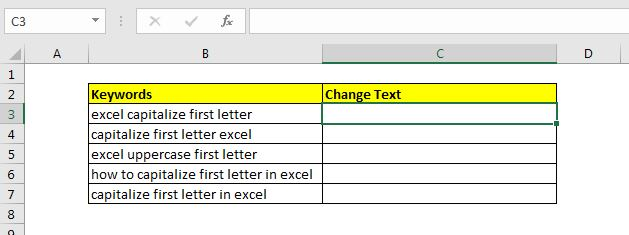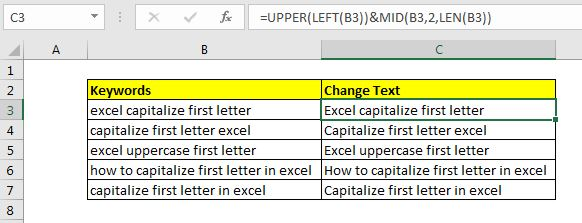# How to Capitalize First Letter in Excel

Sometimes we need to follow the standards of English writings even in Excel (which I am not good at). One of the basic standards of English writing is having the first letter of the sentence in Capital. So how do we capitalize the first letter in each cell?

In this article, we will learn how to make the first letter in uppercase in every cell of Excel.

The generic formula for making the first letter of cell capital is:

 =UPPER(LEFT(text))&MID(text,2,LEN(text))

Text: It is the text of which you want to capitalize the first letter.

Let's get this Formula some job.

Example: Capitalize the first letter of Sentance in ExcelHere, we have a sheet that contains some text values (keyword of this article). Each Cell contains one sentence. We need to capitalize the first letter of each sentence.

Write this formula in cell C3:

 =UPPER(LEFT(B3))&MID(B3,2,LEN(B3))

Hit the enter button. You will have the first letter capitalized in C3. Drag this formula down to make the first letter uppercase in each sentence.How does it work?

It is simple. We have two segments of formula. The first segment (UPPER(LEFT(B3)) capitalizes the first letter of a sentence. How? The LEFT function gets the first letter of the cell. The UPPER function makes it uppercase.

The other segment [MID(B3,2,LEN(B3))] concatenates the rest of the sentence to the uppercase letter.  The LEN function gets the total number of characters and MID function gets the text starting from 2nd character to rest of the text.

Finally, the concatenation operator & concatenates them.

Alternative Method

In this method, we use the RIGHT function instead of the MID function to get the rest of the text as it is.

 =UPPER(LEFT(B3))&RIGHT(B3,LEN(B3)-1)

If you want to capitalize the first letter of each word in the sentence then simply use the PROPER function.

So yeah guys, this how you can capitalize first letter of each cell in excel. I hope it was explanatory and useful to you. If you have any doubts regarding this article or have any other excel/VBA related topic doubt, ask in the comments section below.

Related Articals:

How to Use UPPER function in Excel | The UPPER function in excel is used to capitalize all letters of a string. In this article, we will explore how you can use the UPPER function to do some really important tasks.

Validate only uppercase in data validation | To allow only upper case entries in Excel we can use conditional data validation. By using excel EXACT functions as key function along with function...

How to do Case Sensitive Lookup in Excel | The VLOOKUP function is not a case sensitive function. To lookup case sensitive values we can use EXACT function.

Extract Text From A String In Excel Using Excel’s LEFT And RIGHT Function |  To extract text from strings we normally use RIGHT and LEFT function. These function give a lot of control while manipulating texts.

Popular Articles:

50 Excel Shortcuts to Increase Your Productivity | Get faster at your task. These 50 shortcuts will make you work even faster on Excel.

How to use Excel VLOOKUP Function| This is one of the most used and popular functions of excel that is used to lookup value from different ranges and sheets.

How to use the Excel COUNTIF Function| Count values with conditions using this amazing function. You don't need to filter your data to count specific value. Countif function is essential to prepare your dashboard.

How to Use SUMIF Function in Excel | This is another dashboard essential function. This helps you sum up values on specific conditions.

Terms and Conditions of use

The applications/code on this site are distributed as is and without warranties or liability. In no event shall the owner of the copyrights, or the authors of the applications/code be liable for any loss of profit, any problems or any damage resulting from the use or evaluation of the applications/code.